# Induction without core-size blow-upa.k.a. Large records: anonymous edition

## Edsko de Vries, Adam Gundry – Wednesday, 20 October 2021

all coding compile-time-performance open-source records

An important factor affecting compilation speed and memory requirements is the size of the core code generated by `ghc` from Haskell source modules. Thus, if compilation time is an issue, this is something we should be conscious of and optimize for. In part 1 of this blog post we took an in-depth look at why certain Haskell constructs lead to quadratic blow-up in the generated `ghc` core code, and how the `large-records` library avoids these problems. Indeed, the `large-records` library provides support for records, including support for generic programming, with a guarantee that the generated core is never more than `O(n)` in the number of record fields.

The approach described there does however not directly apply to the case of anonymous records. This is the topic we will tackle in this part 2. Unfortunately, it seems that for anonymous records the best we can hope for is `O(n log n)`, and even to achieve that we need to explore some dark corners of `ghc`. We have not attempted to write a new anynomous records library, but instead consider the problems in isolation; on the other hand, the solutions we propose should be applicable in other contexts as well. Apart from section Putting it all together, the rest of this blog post can be understood without having read part 1.

This work was done on behalf of Juspay; we will discuss the context in a bit more detail in the conclusions.

### Recap: The problem of type arguments

Consider this definition of heterogenous lists, which might perhaps form the (oversimplified) basis for an anonymous records library:

``````data HList xs where
Nil  :: HList '[]
(:*) :: x -> HList xs -> HList (x ': xs)``````

If we plot the size of the core code generated by `ghc` for a Haskell module containing only a single `HList` value

``````newtype T (i :: Nat) = MkT Word

type ExampleFields = '[T 00, T 01, .., , T 99]

exampleValue :: HList ExampleFields
exampleValue = MkT 00 :* MkT 01 :* .. :* MkT 99 :* Nil``````

we get an unpleasant surprise: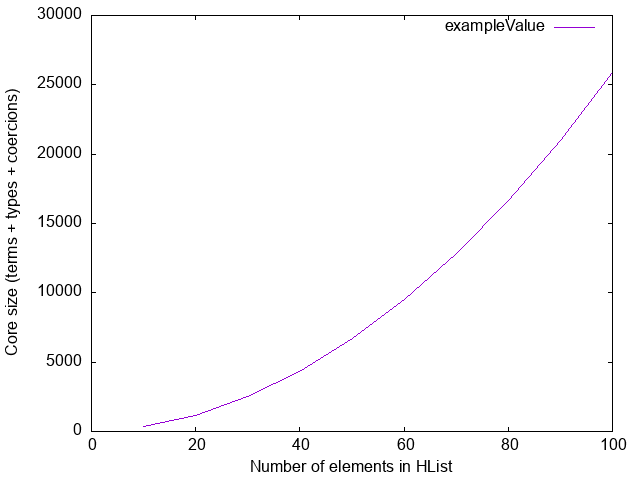The source of this quadratic behaviour is type annotations. The translation of `exampleValue` in `ghc` core is shown below (throughout this blog post I will show “pseudo-core,” using standard Haskell syntax):

``````exampleValue :: HList ExampleFields
exampleValue =
(:*) @(T 00) @'[T 01, T 02, .., T 99] (MkT 00)
\$ (:*) @(T 01) @'[      T 02, .., T 99] (MkT 01)
..
\$ (:*) @(T 99) @'[                    ] (MkT 99)
\$ Nil``````

Every application of the `(:*)` constructor records the names and types of the remaining fields; clearly, this list is `O(n)` in the size of the record, and since there are also `O(n)` applications of `(:*)`, this term is `O(n²)` in the number of elements in the `HList`.

We covered this in detail in part 1. In the remainder of this part 2 we will not be concerned with values of `HList` (`exampleValue`), but only with the type-level indices (`ExampleFields`).

### Instance induction considered harmful

A perhaps surprising manifestation of the problem of quadratic type annotations arises for certain type class dictionaries. Consider this empty class, indexed by a type-level list, along with the customary two instances, for the empty list and an inductive instance for non-empty lists:

``````class EmptyClass (xs :: [Type])

instance EmptyClass '[]
instance EmptyClass xs => EmptyClass (x ': xs)

requireEmptyClass :: EmptyClass xs => Proxy xs -> ()
requireEmptyClass _ = ()``````

Let’s plot the size of a module containing only a single usage of this function:

``````requiresInstance :: ()
requiresInstance = requireEmptyClass (Proxy @ExampleFields)``````

Again, we plot the size of the module against the number of record fields (number of entries in `ExampleFields`):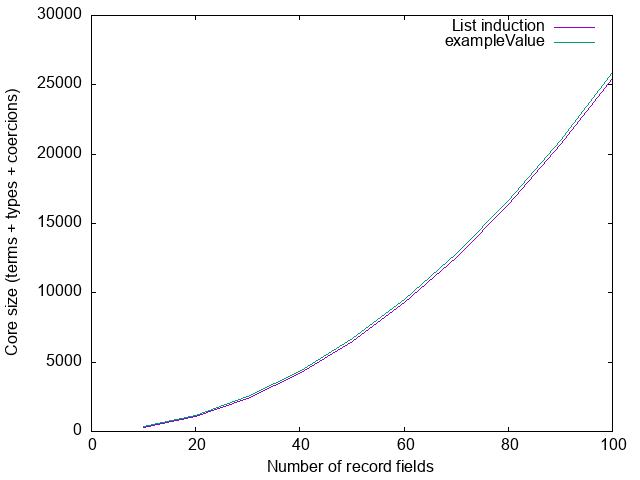The size of this module is practically identical to the size of the module containing `exampleValue`, because at the core level they actually look very similar. The translation of `requiresEmptyClass` looks something like like this:

``````d00  :: EmptyClass '[T 00, T 01, .., T 99]
d01  :: EmptyClass '[      T 01, .., T 99]
..
d99  :: EmptyClass '[                T 99]
dNil :: EmptyClass '[                    ]

d00  = fCons @'[T 01, T 02, .., T 09] @(T 00) d01
d01  = fCons @'[      T 02, .., T 99] @(T 01) d02
..
d99  = fCons @'[                    ] @(T 99) dNil
dNil = fNil

requiresInstance :: ()
requiresInstance = requireEmptyClass @ExampleFields d00 (Proxy @ExampleFields)``````

The two `EmptyClass` instances we defined above turn into two functions `fCons` and `fNil`, with types

``````fNil  :: EmptyClass '[]
fCons :: EmptyClass xs -> EmptyClass (x ': xs)``````

which are used to construct dictionaries. These look very similar to the `Nil` and `(:*)` constructor of `HList`, and indeed the problem is the same: we have `O(n)` calls to `fCons`, and each of those records all the remaining fields, which is itself `O(n)` in the number of record fields. Hence, we again have core that is `O(n²)`.

Note that this is true even though the class is empty! It therefore really does not matter what we put inside the class: any induction of this shape will immediately result in quadratic blow-up. This was already implicit in part 1 of this blog post, but it wasn’t as explicit as it perhaps should have been—at least, I personally did not have it as clearly in focus as I do now.

It is true that if both the module defining the instances and the module using the instance are compiled with optimisation enabled, that might all be optimised away eventually, but it’s not that easy to know precisely when we can depend on this. Moreover, if our goal is to improve compilation time (and therefore the development cycle), we anyway typically do not compile with optimizations enabled.

### Towards a solution

For a specific concrete record we can avoid the problem by simply not using induction. Indeed, if we add

``instance {-# OVERLAPPING #-} EmptyClass ExampleFields``

to our module, the size of the compiled module drops from 25,462 AST nodes to a mere 15 (that’s 15 full stop, not 15k!). The `large-records` library takes advantage of this: rather than using induction to define instances, it generates a single instance for each record type, which has constraints for every field in the record. The result is code that is `O(n)` in the size of the record.

The only reason `large-records` can do this, however, is that every record is explicitly declared. When we are dealing with anonymous records such an explicit declaration does not exist, and we have to use induction of some form. An obvious idea suggests itself: why don’t we try halving the list at every step, rather than reducing it by one, thereby reducing the code from `O(n²)` to `O(n log n)`?

Let’s try. To make the halving explicit1, we define a binary `Tree` type2, which we will use promoted to the type-level:

``````data Tree a =
Zero
| One a
| Two a a
| Branch a (Tree a) (Tree a)``````

`EmptyClass` is now defined over trees instead of lists:

``````class EmptyClass (xs :: Tree Type) where

instance EmptyClass 'Zero
instance EmptyClass ('One x)
instance EmptyClass ('Two x1 x2)
instance (EmptyClass l, EmptyClass r) => EmptyClass ('Branch x l r)``````

We could also change `HList` to be parameterized over a tree instead of a list of fields. Ideally this tree representation should be an internal implementation detail, however, and so we instead define a translation from lists to balanced trees:

``````-- Evens [0, 1, .. 9] == [0, 2, 4, 6, 8]
type family Evens (xs :: [Type]) :: [Type] where
Evens '[]            = '[]
Evens '[x]           = '[x]
Evens (x ': _ ': xs) = x ': Evens xs

-- Odds [0, 1, .. 9] == [1, 3, 5, 7, 9]
type family Odds (xs :: [Type]) :: [Type] where
Odds '[]       = '[]
Odds (_ ': xs) = Evens xs

type family ToTree (xs :: [Type]) :: Tree Type where
ToTree '[]       = 'Zero
ToTree '[x]      = 'One x
ToTree '[x1, x2] = 'Two x1 x2
ToTree (x ': xs) = 'Branch x (ToTree (Evens xs)) (ToTree (Odds xs))``````

and then redefine `requireEmptyClass` to do the translation:

``````requireEmptyClass :: EmptyClass (ToTree xs) => Proxy xs -> ()
requireEmptyClass _ = ()``````

The use sites (`requiresInstance`) do not change at all. Let’s measure again: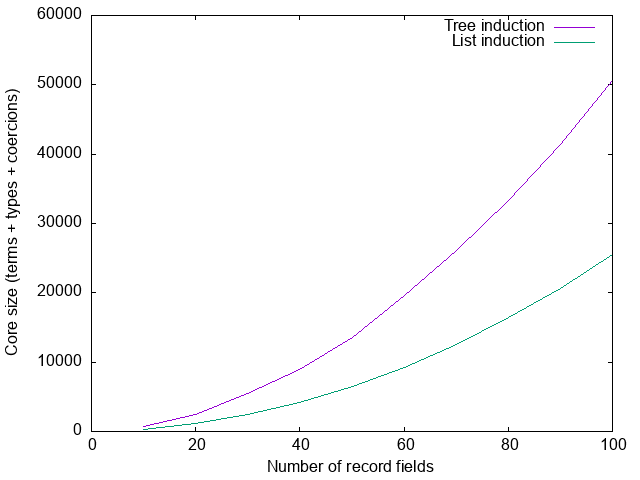Total failure. Not only did we not improve the situation, it got significantly worse.

### What went wrong?

To understand, let’s look at the case for when we have 10 record fields. The generated code looks about as clean as one might hope for:

``````dEx :: EmptyClass (ToTree ExampleFields)
dEx = dTree `cast` <..>

dTree :: EmptyClass (
'Branch
(T 0)
('Branch (T 1) ('Two (T 3) (T 7)) ('Two (T 5) (T 9)))
('Branch (T 2) ('Two (T 4) (T 8)) ('One (T 6)))
)
dTree =
fBranch
@('Branch (T 1) ('Two (T 3) (T 7)) ('Two (T 5) (T 9)))
@('Branch (T 2) ('Two (T 4) (T 8)) ('One (T 6)))
@(T 0)
( fBranch
@('Two (T 3) (T 7))
@('Two (T 5) (T 9))
@(T 1)
(fTwo @(T 3) @(T 7))
(fTwo @(T 5) @(T 9))
)
( -- .. right branch similar
)

requiresInstance :: ()
requiresInstance = requireEmptyClass @ExampleFields dEx (Proxy @ExampleFields)``````

Each recursive call to the dictionary construction function `fBranch` is now indeed happening at half the elements, as intended.

#### The need for a cast

The problem is in the first line:

``dEx = dTree `cast` <..>``

Let’s first understand why we have a cast here at all:

1. The type of the function that we are calling is

``requireEmptyClass :: forall xs. EmptyClass (ToTree xs) => Proxy xs -> ()``

We must pick a value for `xs`: we pick `ExampleFields`.

2. In order to be able to call the function, we must produce evidence (that is, a dictionary) for `EmptyClass (ToTree ExampleFields)`. We therefore have to evaluate `ToTree ...` to `('Branch ...)`; as we do that, we construct a proof `π` that `ToTree ...` is indeed equal to the result `(Branch ...)` that we computed.

3. We now have evidence of `EmptyClass ('Branch ...)`, but we need evidence of `EmptyClass (ToTree ...)`. This is where the cast comes in: we can coerce one to the other given a proof that they are equal; since `π` proves that

``ToTree ... ~ 'Branch ...``

we can construct a proof that

``EmptyClass (ToTree ...) ~ EmptyClass ('Branch ...)``

by appealing to congruence (if `x ~ y` then `T x ~ T y`).

The part in square brackets `<..>` that I elided above is precisely this proof.

#### Proofs

Let’s take a closer look at what that proof looks like. I’ll show a simplified form3, which I hope will be a bit easier to read and in which the problem is easier to spot:

``````  ToTree (T 0) '[T 1, T 2, T 3, T 4, T 5, T 6, T 7, T 8, T 9]
; Branch (T 0)
( Evens (T 1) (T 2) '[T 3, T 4, T 5, T 6, T 7, T 8, T 9]
; Evens (T 3) (T 4) '[T 5, T 6, T 7, T 8, T 9]
; Evens (T 5) (T 6) '[T 7, T 8, T 9]
; Evens (T 7) (T 8) '[T 9]
; Evens (T 9)
; ToTree (T 1) '[T 3, T 5, T 7, T 9]
; Branch (T 1)
( Evens (T 3) (T 5) '[T 7, T 9]
; Evens (T 7) (T 9) '[]
; Evens
; ToTree (T 3) (T 7)
)
( Odds (T 3) '[T 5, T 7, T 9]
; Evens (T 5) (T 7) '[T 9]
; Evens (T 9)
; ToTree (T 5) (T 9)
)
)
( Odds (T 1) '[T 2, T 3, T 4, T 5, T 6, T 7, T 8, T 9]
; .. -- omitted (similar to the left branch)
)``````

The “axioms” in this proof – `ToTree`, `Evens`, etc. – refer to specific cases of our type family definitions. Essentially the proof is giving us a detailed trace of the evaluation of the type families. For example, the proof starts with

``ToTree (T 0) '[T 1, T 2, T 3, T 4, T 5, T 6, T 7, T 8, T 9]``

which refers to the fourth line of the `ToTree` definition

``ToTree (x ': xs) = 'Branch x (ToTree (Evens xs)) (ToTree (Odds xs))``

recording the fact that

``````  ToTree '[T 0, .., T 9]
~ 'Branch (T 0) (ToTree (Evens '[T 1, .. T9])) (ToTree (Odds '[T 1, .. T9]))``````

The proof then splits into two, giving a proof for the left subtree and a proof for the right subtree, and here’s where we see the start of the problem. The next fact that the proof establishes is that

``Evens '[T 1, .. T 9] ~ '[T 1, T 3, T 5, T 7, T 9]``

Unfortunately, the proof to establish this contains a line for every evaluation step:

``````  Evens (T 1) (T 2) '[T 3, T 4, T 5, T 6, T 7, T 8, T 9]
; Evens (T 3) (T 4) '[T 5, T 6, T 7, T 8, T 9]
; Evens (T 5) (T 6) '[T 7, T 8, T 9]
; Evens (T 7) (T 8) '[T 9]
; Evens (T 9)``````

We have `O(n)` such steps, and each step itself has `O(n)` size since it records the remaining list. The coercion therefore has size `O(n²)` at every branch in the tree, leading to an overall coercion also4 of size `O(n²)`.

### Six or half a dozen

So far we defined

``````requireEmptyClass :: EmptyClass (ToTree xs) => Proxy xs -> ()
requireEmptyClass _ = ()``````

and are measuring the size of the core generated for a module containing

``````requiresInstance :: ()
requiresInstance = requireEmptyClass (Proxy @ExampleFields)``````

for some list `ExampleFields`. Suppose we do one tiny refactoring, and make the caller use `ToList` instead; that is, `requireEmptyClass` becomes

``````requireEmptyClass :: EmptyClass t => Proxy t -> ()
requireEmptyClass _ = ()``````

and we now call `ToTree` at the use site instead:

``````requiresInstance :: ()
requiresInstance = requireEmptyClass (Proxy @(ToTree ExampleFields))``````

Let’s measure again: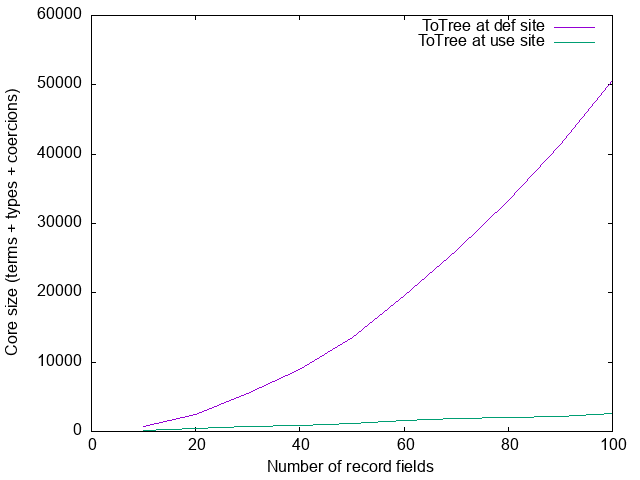The quadratic blow-up disappeared! What happened? Just to add to the confusion, let’s consider one other small refactoring, leaving the definition of `requireEmptyClass` the same but changing the use site to

``requiresInstance = requireEmptyClass @(ToTree ExampleFields) Proxy``

Measuring one more time: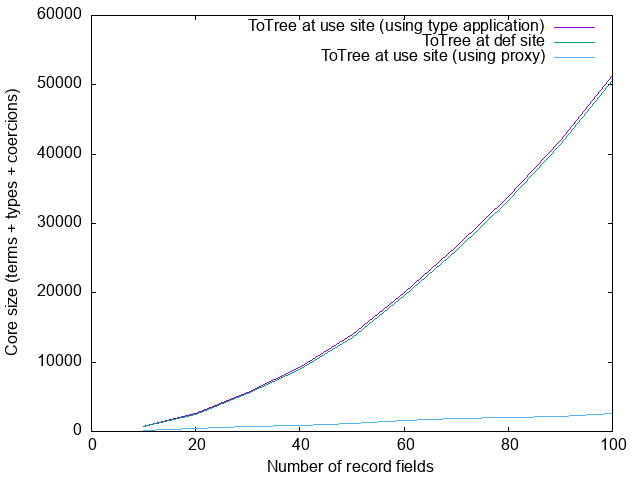Back to blow-up! What’s going on?

#### Roles

We will see in the next section that the difference is due to roles. Before we look at the details, however, let’s first remind ourselves what roles are5. When we define

``newtype Age = MkAge Int``

then `Age` and `Int` are representationally equal but not nominally equal: if we have a function that wants an `Int` but we pass it an `Age`, the type checker will complain. Representational equality is mostly a run-time concern (when do two values have the same representation on the runtime heap?), whereas nominal equality is mostly a compile-time concern. Nominal equality implies representional equality, of course, but not vice versa.

Nominal equality is a very strict equality. In the absence of type families, types are only nominally equal to themselves; only type families introduce additional axioms. For example, if we define

``````type family F (a :: Type) :: Type where
F Int = Bool
F Age = Char``````

then `F Int` and `Bool` are considered to be nominally equal.

When we check whether two types `T a` and `T b` are nominally equal, we must simply check that `a` and `b` are nominally equal. To check whether `T a` and `T b` are representionally equal is however more subtle [Safe zero-cost coercions for Haskell, Fig 3]. There are three cases to consider, illustrated by the following examples:

``````data T1 x = T1
data T2 x = T2 x
data T3 x = T3 (F x)``````

Then

• `T1 a` and `T1 b` are representionally equal no matter the values of `a` and `b`: we say that `x` has a phantom role.
• `T2 a` and `T2 b` are representionally equal if `a` and `b` are: `x` has a representional role.
• `T3 a` and `T3 b` are representionally equal if `a` and `b` are nominally equal: `x` has a nominal role (`T3 Int` and `T3 Age` are certainly not representionally equal, even though `Int` and `Age` are!).

This propagates up; for example, in

``````data ShowType a = ShowType {
showType :: Proxy a -> String
}``````

the role of `a` is phantom, because `a` has a phantom role in `Proxy`. The roles of type arguments are automatically inferred, and usually the only interaction programmers have with roles is through

``coerce :: Coercible a b => a -> b``

where `Coercible` is a thin layer around representational equality. Roles thus remain invisible most of the time—but not today.

#### Proxy versus type argument

Back to the problem at hand. To understand what is going on, we have to be very precise. The function we are calling is

``````requireEmptyClass :: EmptyClass xs => Proxy xs -> ()
requireEmptyClass _ = ()``````

The question we must carefully consider is: what are we instantiating `xs` to? In the version with the quadratic blowup, where the use-site is

``requiresInstance = requireEmptyClass @(ToTree ExampleFields) Proxy``

we are being very explicit about the choice of `xs`: we are instantiating it to `ToTree ExampleFields`. In fact, we are more explicit than we might realize: we are instantiating it to the unevaluated type `ToTree ExampleFields`, not to `('Branch ...)`. Same thing, you might object, and you’d be right—but also wrong. Since we are instantiating it to the unevaluated type, but then build a dictionary for the evaluated type, we must then cast that dictionary; this is precisely the picture we painted in section What went wrong? above.

The more surprising case then is when the use-site is

``requiresInstance = requireEmptyClass (Proxy @(ToTree ExampleFields))``

In this case, we’re leaving `ghc` to figure out what to instantiate `xs` to. It will therefore instantiate it with some fresh variable `𝜏`. When it then discovers that we also have a `Proxy 𝜏` argument, it must unify `𝜏` with `ToTree ExampleFields`. Crucially, when it does so, it instantiates `𝜏` to the evaluated form of `ToTree ExampleFields` (i.e., `Branch ...`), not the unevaluated form. In other words, we’re effectively calling

``requiresInstance = requireEmptyClass @(Branch _ _ _) (Proxy @(ToTree ExampleFields))``

Therefore, we need and build evidence for `EmptyClass (Branch ...)`, and there is no need for a cast on the dictionary.

However, the need for a cast has not disappered: if we instantiate `xs` to `(Branch ...)`, but provide a proxy for `(ToTree ...)`, we need to cast the proxy instead—so why the reduction in core size? Let’s take a look at the equality proof given to the cast:

``````   Univ(phantom phantom <Tree *>
-- (1)    (2)      (3)     (4)
:: ToTree ExampleFields         -- (5)
, 'Branch  (T 0)               -- (6)
('Branch (T 1)
('Two (T 3) (T 7))
('Two (T 5) (T 9)))
('Branch (T 2)
('Two (T 4) (T 8))
('One (T 6)))
)``````

This is it! The entire coercion that was causing us trouble has been replaced basically by a single-constructor “trust me” universal coercion. This is the only coercion that establishes “phantom equality”6. Let’s take a closer look at the coercion:

1. `Univ` indicates that this is a universal coercion.
2. The first occurrence of `phantom` is the role of the equality that we’re establishing.
3. The second occurrence of `phantom` is the provenance of the coercion: what justifies the use of a universal coercion? Here this is `phantom` again (we can use a universal coercion when establishing phantom equality), but this is not always the case; one example is `unsafeCoerce`, which can be used to construct a universal coercion between any two types at all7.
4. When we establish the equality of two types 𝜏1 :: 𝜅1 and 𝜏2 :: 𝜅2, we also need to establish that the two kinds 𝜅1 and 𝜅2 are equal. In our example, both types trivially have the same kind, and so we can just use `<Tree *>`: reflexivity for kind `Tree *`.

Finally, (5) and (6) are the two types that we are proving to be equal to each other.

This then explains the big difference between these two definitions:

``````requiresInstance = requireEmptyClass (Proxy @(ToTree ExampleFields))
requiresInstance = requireEmptyClass @(ToTree ExampleFields) Proxy``````

In the former, we are instantiating `xs` to `(Branch ...)` and we need to cast the proxy, which is basically free due to `Proxy`’s phantom type argument; in the latter, we are instantiating `xs` to `(ToTree ...)`, and we need to cast the dictionary, which requires the full equality proof.

#### Incoherence

If a solution that relies on the precise nature of unification, instantiating type variables to evaluated types rather than unevaluated types, makes you a bit uneasy—I would agree. Moreover, even if we did accept that as unpleasant but necessary, we still haven’t really solved the problem. The issue is that

``````requireEmptyClass :: EmptyClass xs => Proxy xs -> ()
requireEmptyClass _ = ()``````

is a function we cannot abstract over: the moment we define something like

``````abstracted :: forall xs. EmptyClass (ToTree xs) => Proxy xs -> ()
abstracted _ = requireEmptyClass (Proxy @(ToTree xs))``````

in an attempt to make that `ToTree` call a responsibility of a library instead of use sites (after all, we wanted the tree representation to be an implementation detail), we are back to the original problem.

Let’s think a little harder about these roles. We finished the previous section with a remark that “(..) we need to cast the dictionary, which requires the full equality proof.” But why is that? When we discussed roles above, we saw that the `a` type parameter in

``````data ShowType a = ShowType {
showType :: Proxy a -> String
}``````

has a phantom role; yet, when we define a type class

``````class ShowType a where
showType :: Proxy a -> String``````

(which, after all, is much the same thing), `a` is assigned a nominal role, not a phantom role. The reason for this is that `ghc` insists on coherence (see Safe zero-cost coercions for Haskell, section 3.2, Preserving class coherence). Coherence simply means that there is only ever a single instance of a class for a specific type; it’s part of how ghc prevents ambiguity during instance resolution. We can override the role of the `ShowType` dictionary and declare it to be phantom

``type role ShowType phantom``

but we lose coherence:

``````data ShowTypeDict a where
ShowTypeDict :: ShowType a => ShowTypeDict a

showWithDict :: Proxy a -> ShowTypeDict a -> String
showWithDict p ShowTypeDict = showType p

instance ShowType Int where showType _ = "Int"

showTypeInt :: ShowTypeDict Int
showTypeInt = ShowTypeDict

oops :: String
oops = showWithDict (Proxy @Bool) (coerce showTypeInt)``````

This means that the role annotation for `ShowType` requires the `IncoherentInstances` language pragma (there is currently no class-level pragma).

#### Solving the problem

Despite the problem with roles and potential incoherence discussed in the previous section, role annotations on classes cannot make instance resolution ambiguous or result in runtime type errors or segfaults. We do have to be cautious with the use of `coerce`, but we can shield the user from this through careful module exports. Indeed, we have used role annotations on type classes to our advantage before.

Specifically, we can redefine our `EmptyClass` as an internal (not-exported) class as follows:

``````class EmptyClass' (xs :: Tree Type) -- instances as before
type role EmptyClass' phantom``````

then define a wrapper class that does the translation from lists to trees:

``````class    EmptyClass' (ToTree xs) => EmptyClass (xs :: [Type])
instance EmptyClass' (ToTree xs) => EmptyClass (xs :: [Type])

requireEmptyClass :: EmptyClass xs => Proxy xs -> ()
requireEmptyClass _ = ()``````

Now the translation to a tree has become an implementation detail that users do not need to be aware of, whilst still avoiding (super)quadratic blow-up in core: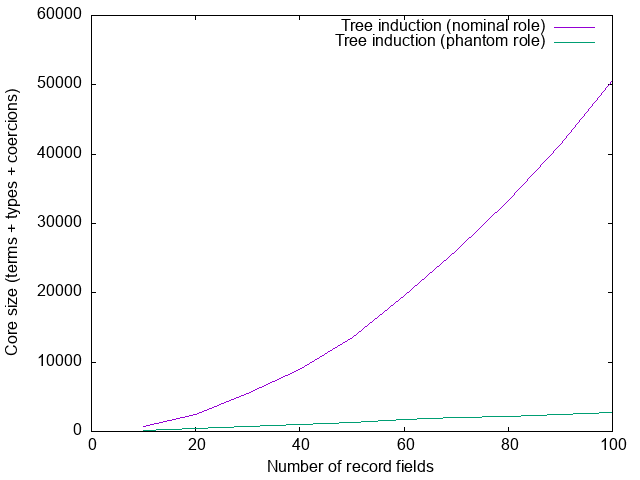### Constraint families

There is one final piece to the puzzle. Suppose we define a type family mapping types to constraints:

``type family CF (a :: Type) :: Constraint``

In the next session we will see a non-contrived example of this; for now, let’s just introduce a function that depends on `CF a` for no particular reason at all:

``````withCF :: CF a => Proxy a -> ()
withCF _ = ()``````

Finally, we provide an instance for `HList`:

``type instance CF (HList xs) = EmptyClass (ToTree xs)``

Now let’s measure the size of the core generated for a module containing a single call

``````satisfyCF :: ()
satisfyCF = withCF (Proxy @(HList ExampleFields))``````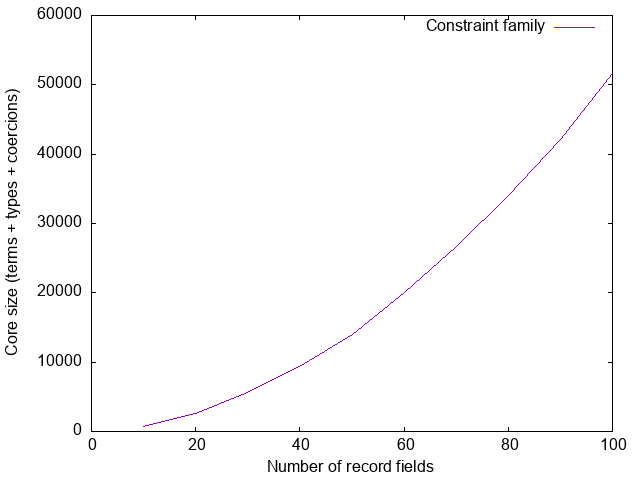Sigh.

#### Shallow thinking

In section Proxy versus type argument above, we saw that when we call

``requireEmptyClass @(Branch _ _ _) (Proxy @(ToTree ExampleFields))``

we construct a proof `π :: Proxy (ToTree ...) ~ Proxy (Branch ...)`, which then gets simplified. We didn’t spell out in detail how that simplification happens though, so let’s do that now.

As mentioned above, the type checker always works with nominal equality. This means that the proof constructed by the type checker is actually `π :: Proxy (ToTree ...) ~N Proxy (Branch ...)`. Whenever we cast something in core, however, we want a proof of representational equality. The coercion language has an explicit constructor for this8, so we could construct the proof

``sub π :: Proxy (ToTree ...) ~R Proxy (Branch ...)``

However, the function that constructs this proof first takes a look: if it is a constructing an equality proof `T π'` (i.e., an appeal to congruence for type `T`, applied to some proof `π'`), where `T` has an argument with a phantom role, it replaces the proof (`π'`) with a universal coercion instead. This also happens when we construct a proof that `EmptyClass (ToTree ...) ~R EmptyClass (Branch ...)` (like in section Solving the problem), provided that the argument to `EmptyClass` is declared to have a phantom role.

Why doesn’t that happen here? When we call function `withCF` we need to construct evidence for `CF (HList ExampleFields)`. Just like before, this means we must first evaluate this to `EmptyClass (Branch ..)`, resulting in a proof of nominal equality `π :: CF .. ~N EmptyClass (Branch ..)`. We then need to change this into a proof of representational equality to use as an argument to `cast`. However, where before `π` looked like `Proxy π'` or `EmptyClass π'`, we now have a proof that looks like

``````  D:R:CFHList <'[T 0, T 1, .., T 9]>_N
; EmptyClass π'``````

which first proves that `CF (HList ..) ~ EmptyClass (ToTree ..)`, and only then proves that `EmptyClass (ToTree ..) ~ EmptyClass (Branch ..)`. The function that attempts the proof simplification only looks at the top-level of the proof, and therefore does not notice an opportunity for simplification here and simply defaults to using `sub π`.

The function does not traverse the entire proof because doing so at every point during compilation could be prohibitively expensive. Instead, it does cheap checks only, leaving the rest to be cleaned up by coercion optimization. Coercion optimization is part of the “very simple optimizer,” which runs even with `-O0` (unless explicitly disabled with `-fno-opt-coercion`). In this particular example, coercion optimization will replace the proof (`π'`) by a universal coercion, but it will only do so later in the compilation pipeline; better to reduce the size of the core sooner rather than later. It’s also not entirely clear if it will always be able to do so, especially also because the coercion optimiser can make things significantly worse in some cases, and so it may be scaled back in the future. I think it’s preferable not to depend on it.

#### Avoiding deep normalization

As we saw, when we call

``withCF (Proxy @(HList ExampleFields))``

the type checker evaluates `CF (HList ExampleFields)` all the way to `EmptyClass (Branch ...)`: although there is ghc ticket proposing to change this, at present whenever `ghc` evaluates a type, it evaluates it all the way. For our use case this is frustrating: if `ghc` were to rewrite `CF (HList ..)` to `ExampleFields (ToTree ..)` (with a tiny proof: just one rule application), we would then be back where we were in section Solving the problem, and we’d avoid the quadratic blow-up. Can we make `ghc` stop evaluating earlier? Yes, sort of. If instead of

``type instance CF (HList xs) = EmptyClass (ToTree xs)``

we say

``````class    EmptyClass (ToTree xs) => CF_HList xs
instance EmptyClass (ToTree xs) => CF_HList xs

type instance CF (HList xs) = CF_HList xs``````

then `CF (HList xs)` simply gets rewritten (in one step) to `CF_HList xs`, which contains no further type family applications. Now the type checker needs to check that `CF_HList ExampleFields` is satisfied, which will match the above instance, and hence it must check that `EmptyClass (ToTree ExampleFields)` is satisfied. Now, however, we really are back in the situation from section Solving the problem, and the size of our core looks good again: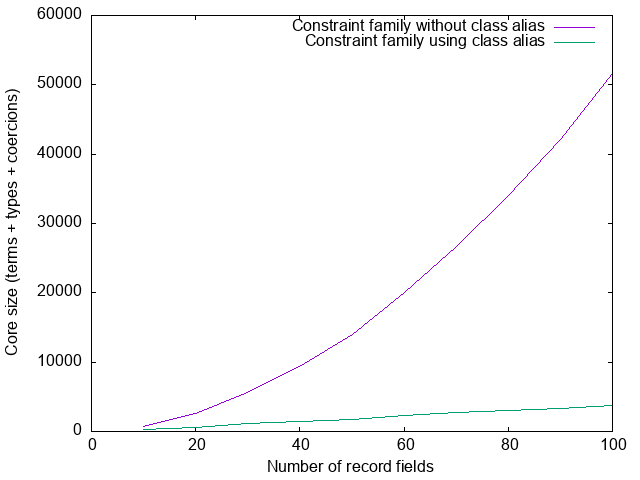### Putting it all together: `Generic` instance for `HList`

Let’s put theory into practice and give a `Generic` instance for `HList`, of course avoiding quadratic blow-up in the process. We will use the `Generic` class from the `large-records` library, discussed at length in part 1.

The first problem we have to solve is that when giving a `Generic` instance for some type `a`, we need to choose a constraint

``Constraints a c :: (Type -> Constraint) -> Constraint``

such that when `Constraints a c` is satisfied, we can get a type class dictionary for `c` for every field in the record:

``````class Generic a where
-- | @Constraints a c@ means "all fields of @a@ satisfy @c@"
type Constraints a (c :: Type -> Constraint) :: Constraint

-- | Construct vector of dictionaries, one for each field of the record
dict :: Constraints a c => Proxy c -> Rep (Dict c) a

-- .. other class members ..``````

Recall that `Rep (Dict c) a` is a vector containing a `Dict c x` for every field of type `x` in the record. Since we are avoiding heterogenous data structures (due to the large type annotations), we effectively need to write a function

``hlistDict :: Constraints a c => Proxy a -> [Dict c Any]``

Let’s tackle this in quite a general way, so that we can reuse what we develop here for the next problem as well. We have a type-level list of types of the elements of the `HList`, which we can translate to a type-level tree of types. We then need to reflect this type-level tree to a term-level tree with values of some type `f Any` (in this example, `f ~ Dict c`). We will delegate reflection of the values in the tree to a separate class:

``````class ReflectOne (f :: Type -> Type) (x :: Type) where
reflectOne :: Proxy x -> f Any

class ReflectTree (f :: Type -> Type) (xs :: Tree Type) where
reflectTree :: Proxy xs -> Tree (f Any)

type role ReflectTree nominal phantom -- critical!``````

Finally, we can then flatten that tree into a list, in such a way that it reconstructs the order of the list (i.e., it’s a term-level inverse to the `ToTree` type family):

``treeToList :: Tree a -> [a]``

The instances for `ReflectTree` are easy. For example, here is the instance for `One`:

``````instance ReflectOne f x => ReflectTree f ('One x) where
reflectTree _ = One (reflectOne (Proxy @x))``````

The other instances for `ReflectTree` follow the same structure (the full source code can be found in the `large-records` test suite). It remains only to define a `ReflectOne` instance for our current specific use case:

``````instance c a => ReflectOne (Dict c) (a :: Type) where
reflectOne _ = unsafeCoerce (Dict :: Dict c a)``````

With this definition, a constraint `ReflectTree (Dict c) (ToTree xs)` for a specific list of `xs` will result in a `c x` constraint for every `x` in `xs`, as expected. We are now ready to give a partial implementation of the `Generic` class:

``````hlistDict :: forall c (xs :: [Type]).
ReflectTree (Dict c) (ToTree xs)
=> Proxy xs -> [Dict c Any]
hlistDict _ = treeToList \$ reflectTree (Proxy @(ToTree xs))

class    ReflectTree (Dict c) (ToTree xs) => Constraints_HList xs c
instance ReflectTree (Dict c) (ToTree xs) => Constraints_HList xs c

instance Generic (HList xs) where
type Constraints (HList xs) = Constraints_HList xs
dict _ = Rep.unsafeFromListAny \$ hlistDict (Proxy @xs)``````

The other problem that we need to solve is that we need to construct field metadata for every field in the record. Our (over)simplified “anonymous record” representation does not have field names, so we need to make them up from the type names. Assuming some type family

``type family ShowType (a :: Type) :: Symbol``

we can construct this metadata in much the same way that we constructed the dictionaries:

``````instance KnownSymbol (ShowType a) => ReflectOne FieldMetadata (a :: Type) where
reflectOne _ = FieldMetadata (Proxy @(ShowType a)) FieldLazy

instance ReflectTree FieldMetadata (ToTree xs) => Generic (HList xs) where
recordName          = "Record"
, recordConstructor   = "MkRecord"
, recordSize          = length fields
}
where
fields = treeToList \$ reflectTree (Proxy @(ToTree xs))``````

The graph below compares the size of the generated core between a straight-forward integration with `generics-sop` and two integrations with `large-records`, one in which the `ReflectTree` parameter has its inferred `nominal` role, and one with the `phantom` role override: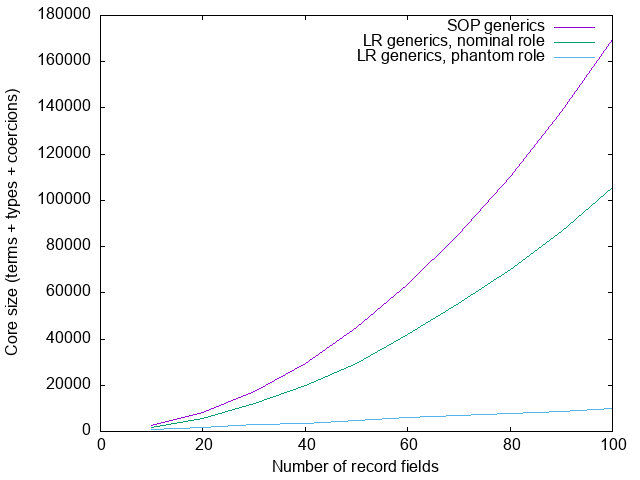Both the `generics-sop` integration and the nominal `large-records` integration are `O(n²)`. The constant factors are worse for `generics-sop`, however, because it suffers from both the problems described in part 1 of this blog as well as the problems described in this part 2. The nominal `large-records` integration only suffers from the problems described in part 2, which are avoided by the `O(n log n)` phantom integration.

We considered a lot of deeply technical detail in this blog post, but I think it can be condensed into two simple rules. To reduce the size of the generated core code:

1. Use instance induction only with balanced data structures.
2. Use expensive type families only in phantom contexts.

where “phantom context” is short-hand for “as an argument to a datatype, where that argument has a phantom role.” The table below summarizes the examples we considered in this blog post.

Don’t Do
`instance C xs => instance C (x ': xs)` `instance (C l, C r) => instance C ('Branch l r)`
`foo @(F xs) Proxy` `foo (Proxy @(F xs))`
`type role Cls nominal` `type role Cls phantom`
Use with caution: requires `IncoherentInstances`
`type instance F a = Expensive a` `type instance F a = ClassAlias a`
(for `F a :: Constraint`)

### Conclusions

This work was done in the context of trying to improve the compilation time of Juspay’s code base. When we first started analysing why Juspay was suffering from such long compilation times, we realized that a large part of the problem was due to the large core size generated by `ghc` when using large records, quadratic in the number of record fields. We therefore developed the `large-records` library, which offers support for records which guarantees to result in core code that is linear in the size of the record. A first integration attempt showed that this improved compilation time by roughly 30%, although this can probably be tweaked a little further.

As explained in MonadFix’s blog post Transpiling a large PureScript codebase into Haskell, part 2: Records are trouble, however, some of the records in the code base are anonymous records, and for these the `large-records` library is not (directly) applicable, and instead, `MonadFix` is using their own library `jrec`.

An analysis of the integration with `large-records` revealed however that these large anonymous records are causing similar problems as the named records did. Amongst the 13 most expensive modules (in terms of compilation time), 5 modules suffered from huge coercions, with less extreme examples elsewhere in the codebase. In one particularly bad example, one function contained coercion terms totalling nearly 2,000,000 AST nodes! (The other problem that stood out was due to large enumerations, not something I’ve thought much about yet.)

We have certainly not resolved all sources of quadratic core code for anonymous records in this blog post, nor have we attempted to integrate these ideas in `jrec`. However, the generic instance for anonymous records (using `generics-sop` generics) was particularly troublesome, and the ideas outlined above should at least solve that problem.

In general the problem of avoiding generating superlinear core is difficult and multifaceted:

Fortunately, `ghc` gives us just enough leeway that if we are very careful we can avoid the problem. That’s not a solution, obviously, but at least there are temporary work-arounds we can use.

### Postscript: Pre-evaluating type families

If the evaluation of type families at compile time leads to large proofs, one for every step in the evaluation, perhaps we can improve matters by pre-evaluating these type families. For example, we could define `ToTree` as

``````type family ToTree (xs :: [Type]) :: Tree Type where
ToTree '[]                       = 'Zero
ToTree '[x0]                     = 'One x0
ToTree '[x0, x1]                 = 'Two x0 x1
ToTree '[x0, x1, x2]             = 'Branch x0 ('One x1)    ('One x2)
ToTree '[x0, x1, x2, x3]         = 'Branch x0 ('Two x1 x3) ('One x2)
ToTree '[x0, x1, x2, x3, x4]     = 'Branch x0 ('Two x1 x3) ('Two x2 x4)
ToTree '[x0, x1, x2, x3, x4, x5] = 'Branch x0 ('Branch x1 ('One x3) ('One x5)) ('Two x2 x4)
...``````

perhaps (or perhaps not) with a final case that defaults to regular evaluation. Now `ToTree xs` can evaluate in a single step for any of the pre-computed cases. Somewhat ironically, in this case we’re better off without phantom contexts: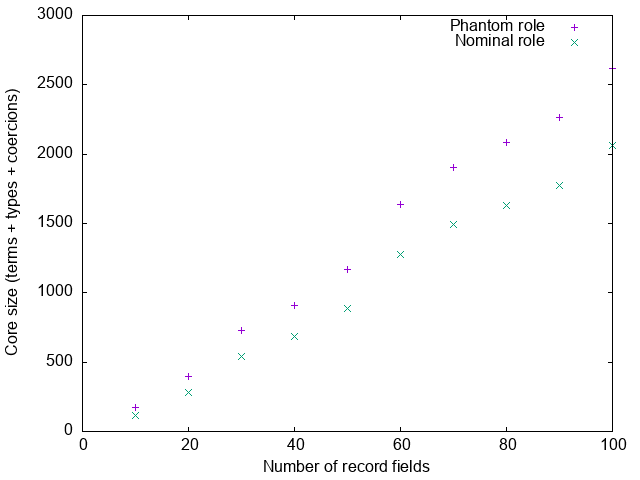The universal coercion is now larger than the regular proof, because it records the full right-hand-side of the type family:

``````Univ(phantom phantom <Tree *>
:: 'Branch
(T 0)
('Branch (T 1) ('Two (T 3) (T 7)) ('Two (T 5) (T 9)))
('Branch (T 2) ('Two (T 4) (T 8)) ('One (T 6)))
, ToTree '[T 0, T 1, T 2, T 3, T 4, T 5, T 6, T 7, T 8, T 9]))``````

The regular proof instead only records the rule that we’re applying:

``D:R:ToTree <T 0> <T 1> <T 2> <T 3> <T 4> <T 5> <T 6> <T 7> <T 8> <T 9>``

Hence, the universal coercion is `O(n log n)`, whereas the regular proof is `O(n)`. Incidentally, this is also the reason why `ghc` doesn’t just always use universal coercions; in some cases the universal coercion can be significantly larger than the regular proof (the `Rep` type family of `GHC.Generics` being a typical example). The difference between `O(n)` and `O(n log n)` is of course significantly less important than the difference between `O(n log n)` and `O(n²)`, especially since we anyway still have other `O(n log n)` factors, so if we can precompute type families like this, perhaps we can be less careful about roles.

However, this is only a viable option for certain type families. A full-blown anonymous records library will probably need to do quite a bit of type-level computation on the indices of the record. For example, the classical extensible records theory by Gaster and Jones depends crucially on a predicate `lacks` that checks that a particular field is not already present in a record. We might define this as

``````type family Lacks (x :: k) (xs :: [k]) :: Bool where
Lacks x '[]       = 'True
Lacks x (x ': xs) = 'False
Lacks x (y ': xs) = Lacks x xs``````

It’s not clear to me how to pre-compute this type family in such a way that the right-hand side can evaluate in `O(1)` steps, without the type family needing `O(n²)` cases. We might attempt

``````type family Lacks (x :: k) (xs :: [k]) :: Bool where
-- 0
Lacks x '[] = 'True

-- 1
Lacks x '[x] = 'False
Lacks x '[y] = 'True

-- 2
Lacks x '[x  , y2] = 'False
Lacks x '[y1 , x ] = 'False
Lacks x '[y1 , y2] = 'True

-- 3
Lacks x '[x,  y2 , y3] = 'False
Lacks x '[y1, x  , y3] = 'False
Lacks x '[y1, y2 , x ] = 'False
Lacks x '[y1, y2 , y3] = 'True

-- etc``````

but even if we only supported records with up to 200 fields, this would require over 20,000 cases. It’s tempting to try something like

``````type family Lacks (x :: k) (xs :: [k]) :: Bool where
Lacks x []           = True
Lacks x [y0]         = (x != y0)
Lacks x [y0, y1]     = (x != y0) && (x != y1)
Lacks x [y0, y1, y2] = (x != y0) && (x != y1) && (x != y2)
..``````

but that is not a solution (even supposing we had such an `!=` operator): the right hand side still has `O(n)` operations, and so this would still result in proofs of `O(n)` size, just like the non pre-evaluated `Lacks` definition. Type families such as `Sort` would be more difficult still. Thus, although precomputation can in certain cases help avoid large proofs, and it’s a useful technique to have in the arsenal, I don’t think it’s a general solution.

##### Footnotes

1. The introduction of the `Tree` datatype is not strictly necessary. We could instead work exclusively with lists, and use `Evens`/`Odds` directly to split the list in two at each step. The introduction of `Tree` however makes this structure more obvious, and as a bonus leads to smaller core, although the difference is a constant factor only.↩︎

2. This `Tree` data type is somewhat non-standard: we have values both at the leaves and in the branches, and we have leaves with zero, one or two values. Having values in both branches and leaves reduces the number of tree nodes by one half (leading to smaller core), which is an important enough improvement to warrant the minor additional complexity. The `Two` constructor only reduces the size the tree roughly by a further 4%, so not really worth it in general, but it’s useful for the sake of this blog post, as it keeps examples of trees a bit more manageable.↩︎

3. Specifically, I made the following simplifications to the actual coercion proof:
1. Drop the use of `Sym`, replacing `Sym (a ; .. ; c)` by `(Sym c ; .. ; Sym a)` and `Sym c` by simply `c` for atomic axioms `c`.
2. Appeal to transitivity to replace `a ; (b ; c)` by `a ; b ; c`
3. Drop all explicit use of congruence, replacing `T c` by simply `c`, whenever `T` has a single argument.
4. As a final step, reverse the order of the proof; in this case at least, `ghc` seemed to reason backwards from the result of the type family application, but it’s more intuitive to reason forwards instead.↩︎

4. Roles were first described in Generative Type Abstraction and Type-level Computation. Phantom roles were introduced in Safe zero-cost coercions for Haskell.↩︎

5. Safe zero-cost coercions for Haskell does not talk about this in great detail, but does mention it. See Fig 5, “Formation rules for coercions,” rule `Co_Phantom`, as well as the (extremely brief) section 4.2.2., “Phantom equality relates all types.” The paper does not use the terminology “universal coercion.”↩︎

6. Confusingly the pretty-printer uses a special syntax for a universal unsafe coercion, using `UnsafeCo`instead of `Univ`.↩︎

7. Safe zero-cost coercions for Haskell, section 4.2.1: Nominal equality implies representational equality.↩︎